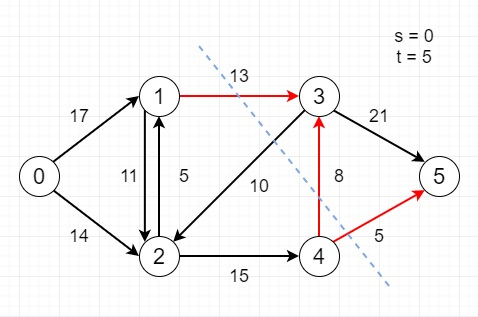# Find minimum s-t cut in a flow network in C++

Suppose we have following flow network. As we know an s-t cut is a cut that requires the source s node and a sink t node to be in different subsets, and it includes edges going from the source set to the sink side. Here the capacity of an s-t cut is represented by the sum of each edge capacity in the cut-set. Here we have to find minimum capacity s-t cut of the given network. Here the expected output is all edges of the minimum cut.

So, if the input is likethen the output will be [(1,3), (4,3), (4,5)]

To solve this, we will follow these steps −

• NODES = 6

• Define a function bfs(), this will take graph, src, sink, array par,

• Define an array vis of size − NODES. and fill with 0

• Define one queue que

• insert src into que

• vis[src] := true and par[src] := -1

• while (que is not empty), do −

• u1 := first element of que

• delete element from que

• for initialize v1 := 0, when v1 < NODES, update (increase v1 by 1), do−

• if vis[v1] is false and graph[u1, v1] > 0, then −

• insert v1 into que

• par[v1] := u1

• vis[v1] := true

• return true when vis[sink] is true

• Define a function dfs(), this will take graph, src, array vis,

• vis[src] := true

• for initialize i := 0, when i < NODES, update (increase i by 1), do−

• if graph[src, i] is non-zero and vis[i] is false, then −

• dfs(graph, i, vis)

• From the main method, do the following −

• Define an array temp_graph and copy graph into it

• Define an array par of size: NODES.

• while bfs(temp_graph, src, sink, par) is true, do −

• path_flow := inf

• for initialize v := sink, when v is not equal to src, update v:=par[v], do −

• u := par[v]

• path_flow := minimum of path_flow and temp_graph[u, v]

• for initialize v := sink, when v is not equal to src, update v:=par[v], do −

• u := par[v]

• temp_graph[u, v] := temp_graph[u, v] - path_flow

• temp_graph[v, u] := temp_graph[v, u] + path_flow

• Define an array vis of size − NODES. and fill with false

• dfs(temp_graph, src, vis)

• for initialize i := 0, when i − NODES, update (increase i by 1), do −

• for initialize j := 0, when j − NODES, update (increase j by 1), do −

• if vis[i] is non-zero and vis[j] is false and graph[i, j] is non-zero, then −

• display (i, j) as edge

• return

## Example (C++)

Let us see the following implementation to get better understanding −

Live Demo

#include <bits/stdc++.h>
using namespace std;
#define NODES 6
int bfs(int graph[NODES][NODES], int src, int sink, int par[]) {
bool vis[NODES];
memset(vis, 0, sizeof(vis));
queue <int> que;
que.push(src);
vis[src] = true;
par[src] = -1;
while (!que.empty()) {
int u1 = que.front();
que.pop();
for (int v1=0; v1<NODES; v1++){
if (vis[v1]==false && graph[u1][v1] > 0) {
que.push(v1);
par[v1] = u1;
vis[v1] = true;
}
}
}
return (vis[sink] == true);
}
void dfs(int graph[NODES][NODES], int src, bool vis[]) {
vis[src] = true;
for (int i = 0; i < NODES; i++)
if (graph[src][i] && !vis[i])
dfs(graph, i, vis);
}
void minCut(int graph[NODES][NODES], int src, int sink) {
int u, v;
int temp_graph[NODES][NODES];
for (u = 0; u < NODES; u++)
for (v = 0; v < NODES; v++)
temp_graph[u][v] = graph[u][v];
int par[NODES];
while (bfs(temp_graph, src, sink, par)){
int path_flow = INT_MAX;
for (v=sink; v!=src; v=par[v]) {
u = par[v];
path_flow = min(path_flow, temp_graph[u][v]);
}
for (v=sink; v != src; v=par[v]) {
u = par[v];
temp_graph[u][v] -= path_flow;
temp_graph[v][u] += path_flow;
}
}
bool vis[NODES];
memset(vis, false, sizeof(vis));
dfs(temp_graph, src, vis);
for (int i = 0; i < NODES; i++)
for (int j = 0; j < NODES; j++)
if (vis[i] && !vis[j] && graph[i][j])
cout << "("<< i << ", " << j << ")" << endl;
return;
}
int main() {
int graph1[NODES][NODES] = {
{0, 17, 14, 0, 0, 0},
{0, 0, 11, 13, 0, 0},
{0, 5, 0, 0, 15, 0},
{0, 0, 9, 0, 0, 21},
{0, 0, 0, 8, 0, 5},
{0, 0, 0, 0, 0, 0}
};
minCut(graph1, 0, 5);
}

## Input

{{0, 17, 14, 0, 0, 0},
{0, 0, 11, 13, 0, 0},
{0, 5, 0, 0, 15, 0},
{0, 0, 9, 0, 0, 21
{0, 0, 0, 8, 0, 5},
{0, 0, 0, 0, 0, 0}};

## Output

(1, 3)
(4, 3)
(4, 5)